# Waste

How many percents are waste from a circular plate with a radius of 1 m from which we cut a square with the highest area?

Result

p =  36.338 %

#### Solution:Leave us a comment of example and its solution (i.e. if it is still somewhat unclear...):Be the first to comment!#### To solve this example are needed these knowledge from mathematics:

Our percentage calculator will help you quickly calculate various typical tasks with percentages. Pythagorean theorem is the base for the right triangle calculator.

## Next similar examples:

1. Cutting circlesFrom the square 1 m side we have to cut the circles with a radius of 10 cm. How many discs we cut and how many percent will be waste?
2. Cutting square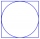From a square with a side of 30 cm, we cut the circle with the highest possible diameter. How many percents of the square content is this circle?
3. Diameters of circlesHow many percent of the area of a larger circle is a smaller circle if the smaller circle has a diameter 120 mm and a larger one has a diameter 300 mm?
4. Round table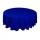Round table with diameter d = 105 cm is coated by square tablecloth with a side length 121 cm. About how many cm is higher center of tablecloth than its cornes?
5. Content area and percentsDetermine what percentage is smaller cube surface, when the surface area of the wall decreases by 25%.
6. Sphere cutsAt what distance from the center intersects sphere with radius R = 56 plane, if the cut area and area of the main sphere circle is in ratio 1/2.
7. Logs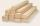The log has diameter 30 cm. What's largest beam with a rectangular cross-section can carve from it?
8. Circular ringSquare with area 16 centimeters square are inscribed circle k1 and described circle k2. Calculate the area of circular ring, which circles k1, k2 form.
9. Circular lawnAround a circular lawn area is 2 m wide sidewalk. The outer edge of the sidewalk is curb whose width is 2 m. Curbstone and the inner side of the sidewalk together form a concentric circles. Calculate the area of the circular lawn and the result round to 1
10. Profit gainIf 5% more is gained by selling an article for Rs. 350 than by selling it for Rs. 340, the cost of the article is:
11. Annular areaThe square with side a = 1 is inscribed and circumscribed by circles. Find the annular area.
12. Common chordTwo circles with radius 17 cm and 20 cm are intersect at two points. Its common chord is long 27 cm. What is the distance of the centers of these circles?
13. Quatrefoil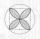Calculate area of the quatrefoil which is inscribed in a square with side 6 cm.
14. SquareCalculate area of the square with diagonal 64 cm.
15. AceThe length of segment AB is 24 cm and the point M and N divided it into thirds. Calculate the circumference and area of this shape.
16. SatinSanusha buys a piece of satin 2.4 m wide. The diagonal length of the fabric is 4m. What is the length of the piece of satin?
17. Square2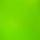Side of the square is a = 6.2 cm, how long is its diagonal?